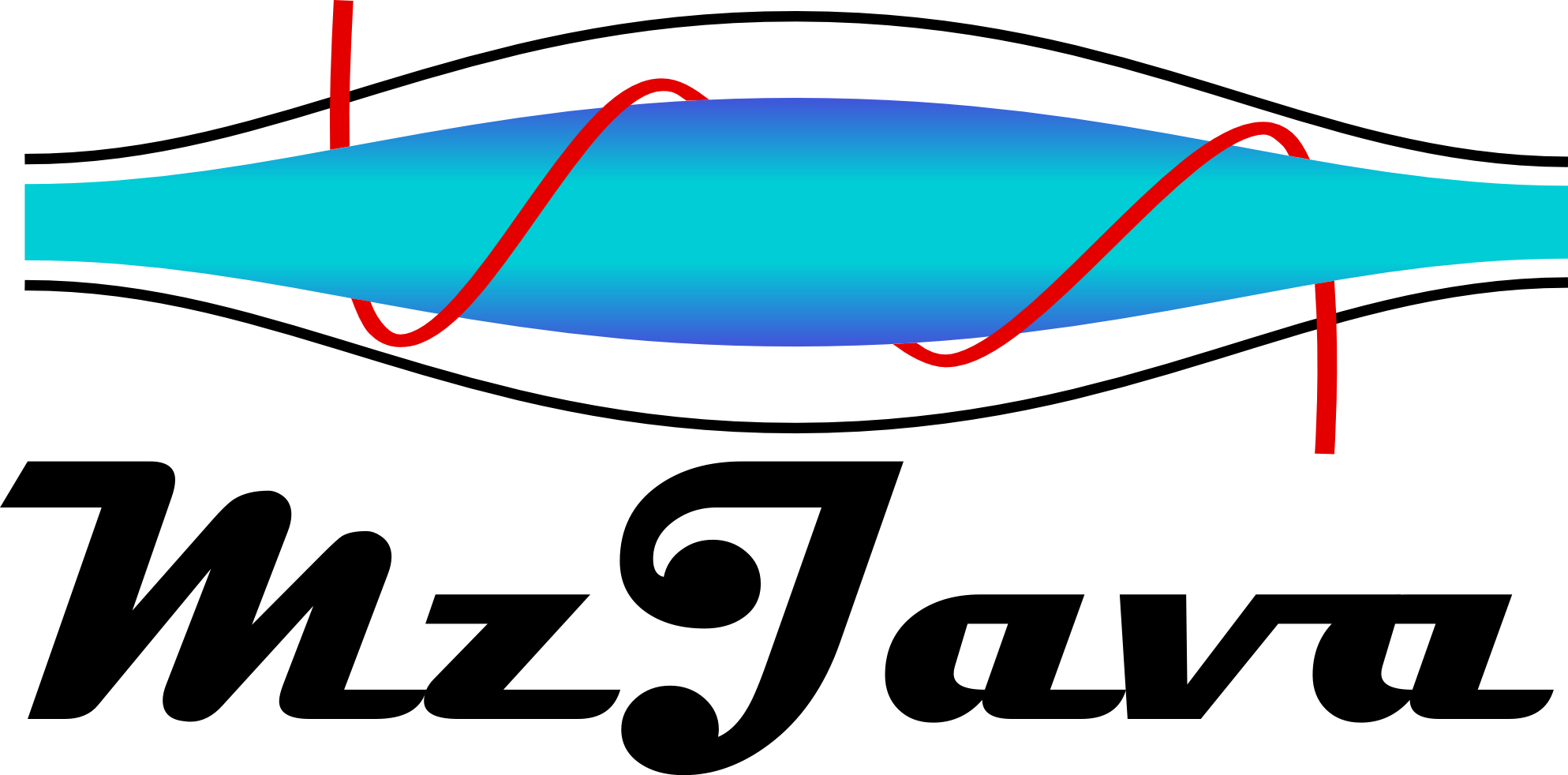# Recipe 1.7 - Creating Modification

## Problem

You want to create Modifications.

## Solution

A Modification is a labeled Mass that can be added to AminoAcidSequence. There are two ways to build an instance of Modification class, using the constructor with a label and a Mass (NumericMass, Composition and NeutralLoss are Masses)

``````
``````

or using the static factory method parseModification from a string with the following format: (label:)?formula

``````
Modification mod = Modification.parseModification("phospho:HPO3");
``````

or the same method without label (in this case the label is the formula)

``````
Modification mod = Modification.parseModification("HPO3");
``````

here is a list of getters

``````
String label = mod.getLabel();
Mass mass = mod.getMass();
double molecularMass = mod.getMolecularMass();
``````